# Detect Replay Attacks in DC Microgrids Using Distributed Watermarking

This example shows how to use distributed watermarking to detect replay attacks in a cyber-physical system (CPS). A DC Microgrid (DCmG) is an interconnected system of distributed generation units (DGU) that represent physical systems and are controlled using current consensus loops responsible for load sharing across multiple units.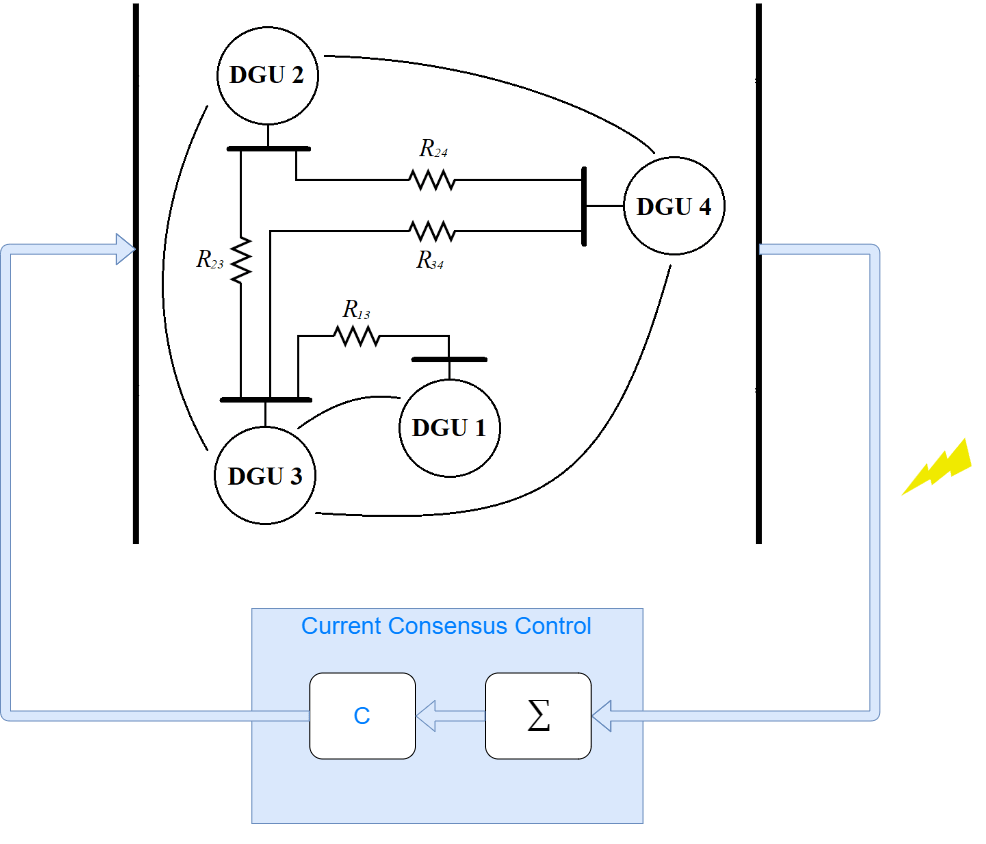This architecture requires the line currents of each DGU to communicate. The communication channel is open to attacks that can inject false information and alter the behavior of the central current consensus loop. One such attack is a replay attack, which delays the signals in the communication channel.

### DC Microgrid Model

In this example, the DCmG consists of two connected distributed generation units modeled using the Average-Value DC-DC Converter (Simscape Electrical) blocks.Set the variables that enable (`1`) or disable (`0`) the different modes. Start with all modes disabled. Use a sample time of 50 microseconds and a simulation duration of 5 seconds.

```enableAttack = 0; enableObserver = 0; enableWatermarking = 0; Ts = 5e-5; stopTime = 5;```

Specify the DGU parameters.

```Vin = 100; Ct = 2.2e-3; Lt = 1.8e-3; Rt = 0.2;```

Specify the power line parameters.

```Lij = 1.8e-6; Rij = 0.05;```

Modify the load current for the first unit to show the voltage and line current transients, as both units share the changed load.

```ILoadInitial = 3; ILoadFinal = 2; tLoadChange = 4;```

The model uses a PID controller for the primary local voltage control of each DGU. Specify parameters for the controllers.

```Vref = 48; Kp = 0.1; Ki = 6.15; Kd = 4.6e-4; N = 2.56e3; Tf = 1/N;```

The current consensus control is based on the weighted sum of normalized individual line currents by the rated capacity of the DGU. Define the capacities and PID control parameters.

```ICapacity_1 = 3; ICapacity_2 = 6; CC_Kp = 0; CC_Ki = 150; CC_Kd = 0;```

Open and simulate the model. You can see the current sharing between the two units at time `t` = 4 s, when the load in the first unit changes.

```Simulink.sdi.view Simulink.sdi.clear open_system("AttackDetectionInDCMicrogrid.slx"); sim("AttackDetectionInDCMicrogrid.slx"); Simulink.sdi.loadView("DCmGCurrentSharingView.mldatx");```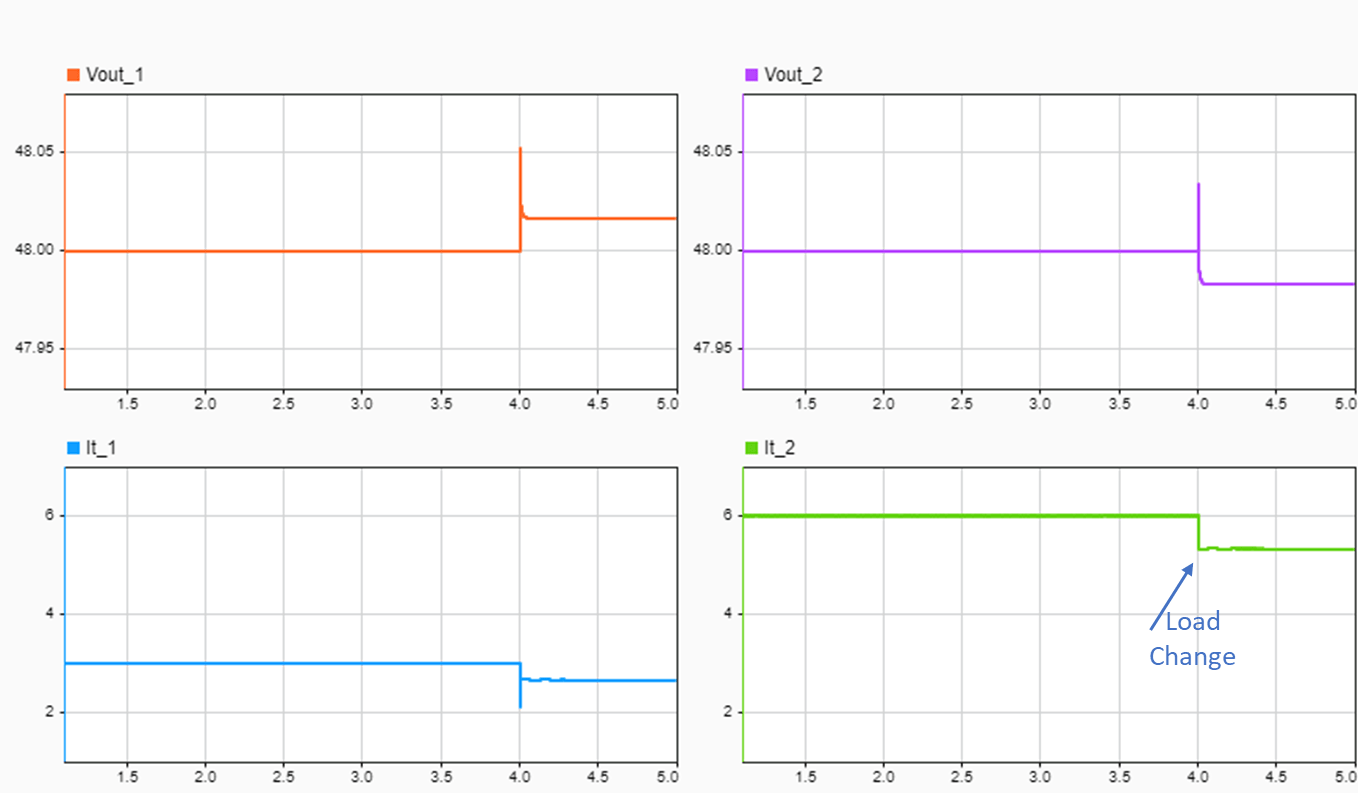The currents in both units (bottom row of the plot) continue to be proportional to the individual rated capacities. The top row of the plot shows the unbalanced voltages.

### Replay Attack

A replay attack is when a malicious agent can observe the signals in the communication channel and then replace current transmitted measurements with delayed recorded observations. This attack delays the signals in the communication channel. It is particularly deceptive when the system is at steady state.

This example uses a replay attack of the following form.

`${\mathit{y}}_{\mathit{a}}\left(\mathit{t}\right)=\mathit{y}\left(\mathit{t}\right)+\beta \left(\mathit{t}-{\mathit{T}}_{\mathit{a}}\right)\left[-\mathit{y}\left(\mathit{t}\right)+\mathit{y}\left(\mathit{t}-{\mathit{t}}_{0}\right)\right]$`

Here, $\beta \left(\mathit{t}-{\mathit{T}}_{\mathit{a}}\right)\text{\hspace{0.17em}}$represents an activation function starting at time ${\mathit{T}}_{\mathit{a}}$ that delays the signal $\mathit{y}\left(\mathit{t}\right)$ by ${\mathit{t}}_{0}$, and ${\mathit{y}}_{\mathit{a}}\left(\mathit{t}\right)$ represents the modified signal.

Enable the attack, and specify the time of attack and the delay the attack introduces in the signal.

```enableAttack = 1; timeOfAttack = 3; attackTimeDelay = 0.1;```

Simulate the model.

```Simulink.sdi.clear sim("AttackDetectionInDCMicrogrid.slx"); Simulink.sdi.loadView("DCmGAttackSimulationView.mldatx");```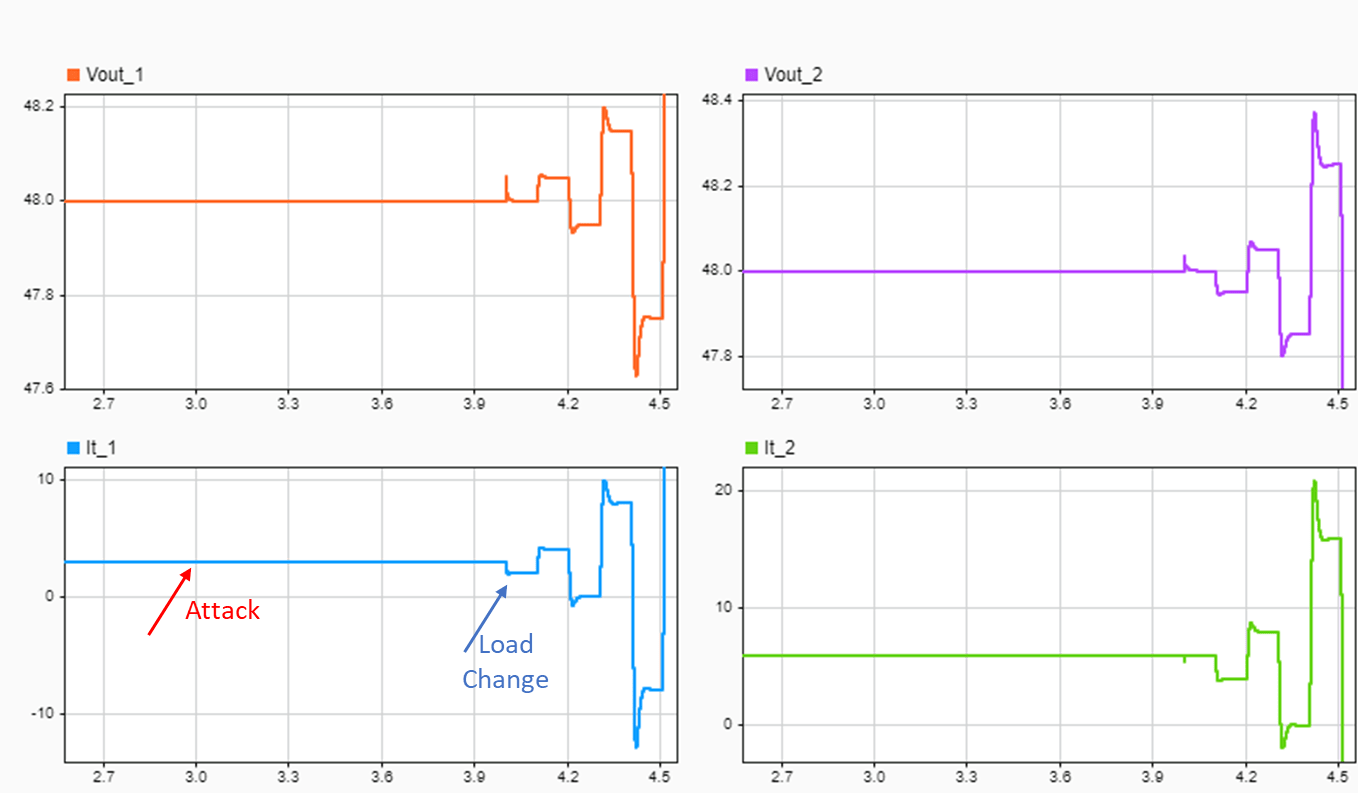This attack results in undesired behavior when the load changes. The current consensus control does not perceive the changes to the line current due to the delay introduced in the communication channel.

The attack occurs from `t` = 3 seconds onwards, but it is not detected while the system is at steady state. The load changes at `t` = 4 seconds and incorrect measurements at the consensus controller result in an unstable system. Uncontrolled line currents (bottom row of the plot) exceed the rated capacity within 300 milliseconds of the load change, damaging the local DGU.

### Watermarking and Attack Detection

Introduce a time-varying signal, unknown to the attacker, as a watermark and use the residuals generated by an observer to detect any modifications or false data injections to the signals in the communication channel.

```enableObserver = 1; enableWatermarking = 1;```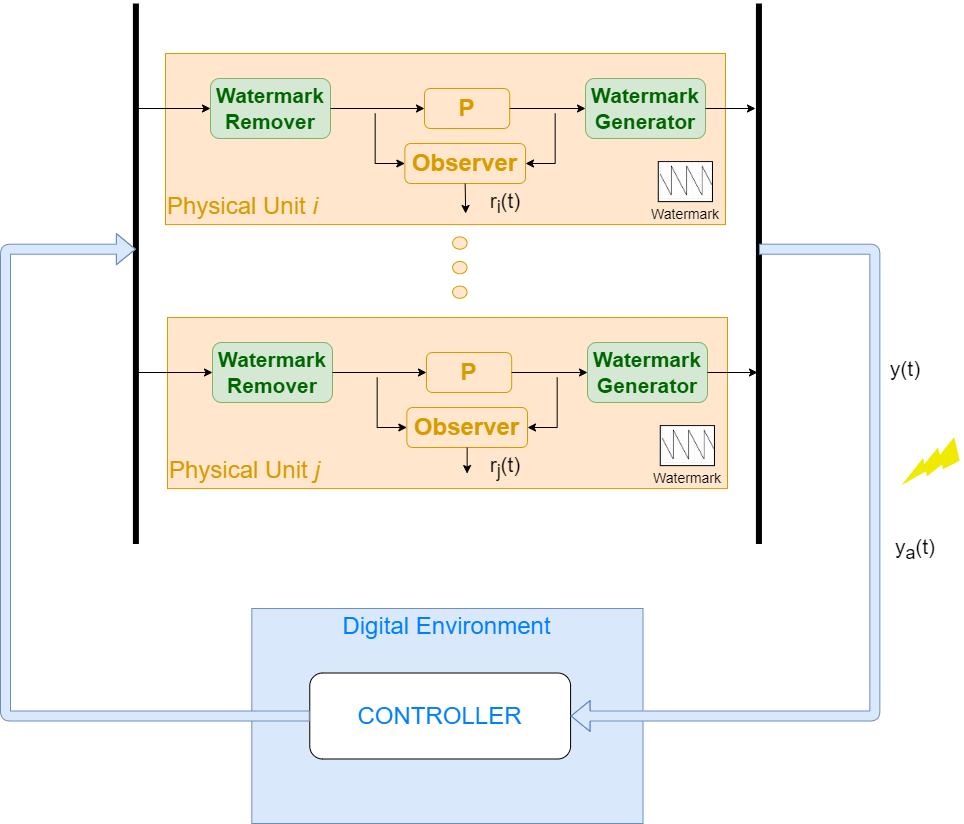The watermark signal is a periodic sawtooth wave, which the `Add Watermark` subsystems in the model inject into the voltage and current measurements communicated to the current consensus controller. The `Remove Watermark` subsystems subtract this signal from the feedback before the subsystems compute the adjustment to the reference voltage and generate the observer residuals. The watermark signal transforms the delay the attack introduces in the signals into a disturbance. Therefore, the system detects an attack when the observer generates a nonzero residual. The amplitude of the watermark signal must be carefully set to allow the detection of a replay attack but not let currents exceed the rated capacity prior to the detection. In this example, the watermark signal amplitude is set to 0.1 and the frequency is set to 2 Hertz.

The model computes the estimates of the model states using a linear Kalman filter and the following model.

`$\begin{array}{l}{\underset{}{\overset{˙}{V}}}_{i}\left(t\right)=\frac{1}{{C}_{ti}}{I}_{ti}\left(t\right)-\frac{1}{{C}_{ti}{R}_{ij}}{V}_{i}\left(t\right)+\frac{1}{{C}_{ti}{R}_{ij}}{V}_{j}\left(t\right)-\frac{1}{{C}_{ti}}{I}_{Li}\left(t\right)\\ {\underset{}{\overset{˙}{I}}}_{ti}\left(t\right)=-\frac{1}{{L}_{ti}}{V}_{i}\left(t\right)-\frac{{R}_{ti}}{{L}_{ti}}{I}_{ti}\left(t\right)+\frac{1}{{L}_{ti}}{V}_{ti}\left(t\right)\end{array}$`

Specify the state-space model parameters.

```At = [-1/(Rij*Ct), 1/Ct; -1/Lt, -Rt/Lt]; Bt = [0; 1/Lt]; Mt = [-1/Ct; 0]; Ht = [1 0]; Bj = [1/(Rij*Ct); 0]; BIl = [-1/Ct; 0]; dguPlant = ss(At,[Bt, Bj, BIl],Ht,0); dguPlant.StateName = {'Vi','Iti'}; dguPlant.InputName = {'Vti','Vj','Ili'}; dguPlant.OutputName = {'Vi'}; dguPlantD = c2d(dguPlant,Ts);```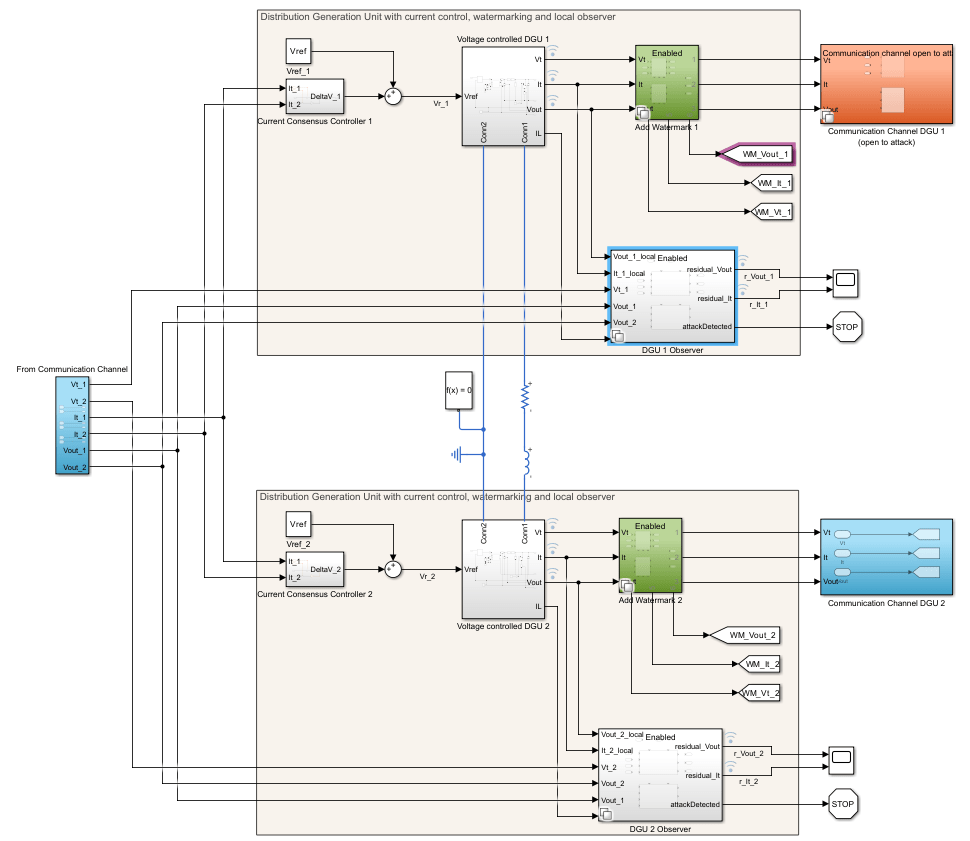Simulate the model.

```Simulink.sdi.clear sim("AttackDetectionInDCMicrogrid.slx"); Simulink.sdi.loadView("DCmGAttackDetectionView.mldatx");```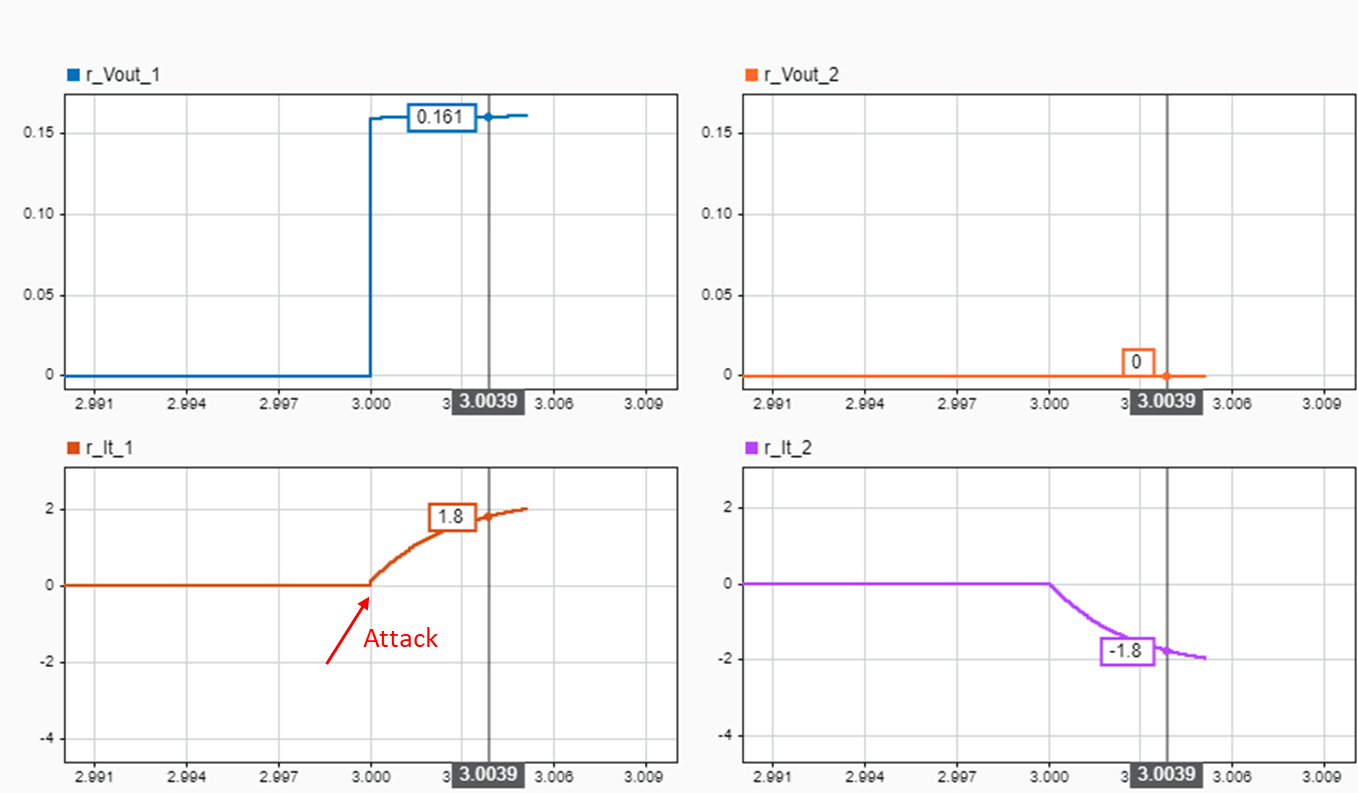The residual plots show the response to the attack at `t` = 3 seconds. The difference between the observed voltage in DGU 1 (top left), and the observed line currents in both DGUs (bottom row) is non-zero and crosses specified thresholds within 4 milliseconds. The difference in voltage of DGU 2 (top right) remains zero, indicating that the signals from DGU 1 were attacked.

You can extend this approach to detect attacks in multiple DGUs and use a threshold to trigger corrective actions, such as isolating the unit that is attacked.

Close the model.

```Simulink.sdi.close close_system('AttackDetectionInDCMicrogrid')```